# ISRO Scientist or Engineer Electronics 2015

Instructions

For the following questions answer them individually

Question 1

# Electron mobility of the following intrinsic elements in descending order is:Question 2

# A sample of Si is doped with $$10^{17}$$ donor atoms/cm$$^3$$. Considering electron mobility in the doped Si 700 cm$$^2$$/V-sec, the approximate resistivity of the doped Si is:Question 3

# Common-base current gain of a p-n-p bipolar transistor is 0.99. The common emitter current gain of the transistor is:Question 4

# The electrical conductivity of a semiconductor increases when a radiation of wavelength shorter than 1000 nm is incident on it. The band gap of the semiconductor is:Question 5

# The voltage across the resistor R is: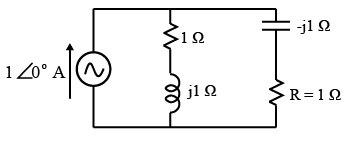Question 6

# The effective resistance faced by the voltage source is: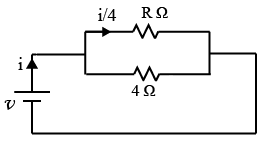Question 7

# Common emitter DC current gain of the transistor is 100. The current through the 10 V Zener diode (assuming Vzs of the transistor is 0.7 V) is: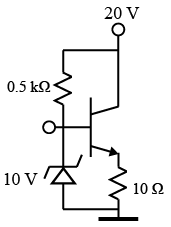Question 8

# The circuit is with an ideal operational amplifier with $$\pm 10 V$$ supply. The output voltage is: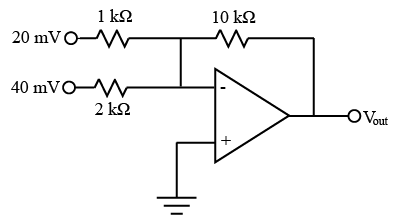Question 9

# A step voltage of 10 V applied to the circuit at t = 0. The current through the resistor R just after t = 0 and at steady state are: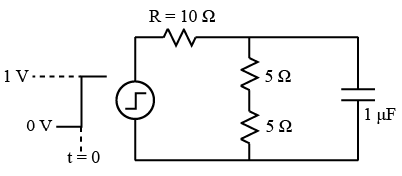Question 10

# Which of the following addressing mode is not usable in XCH instruction of 8051 microcontroller?OR